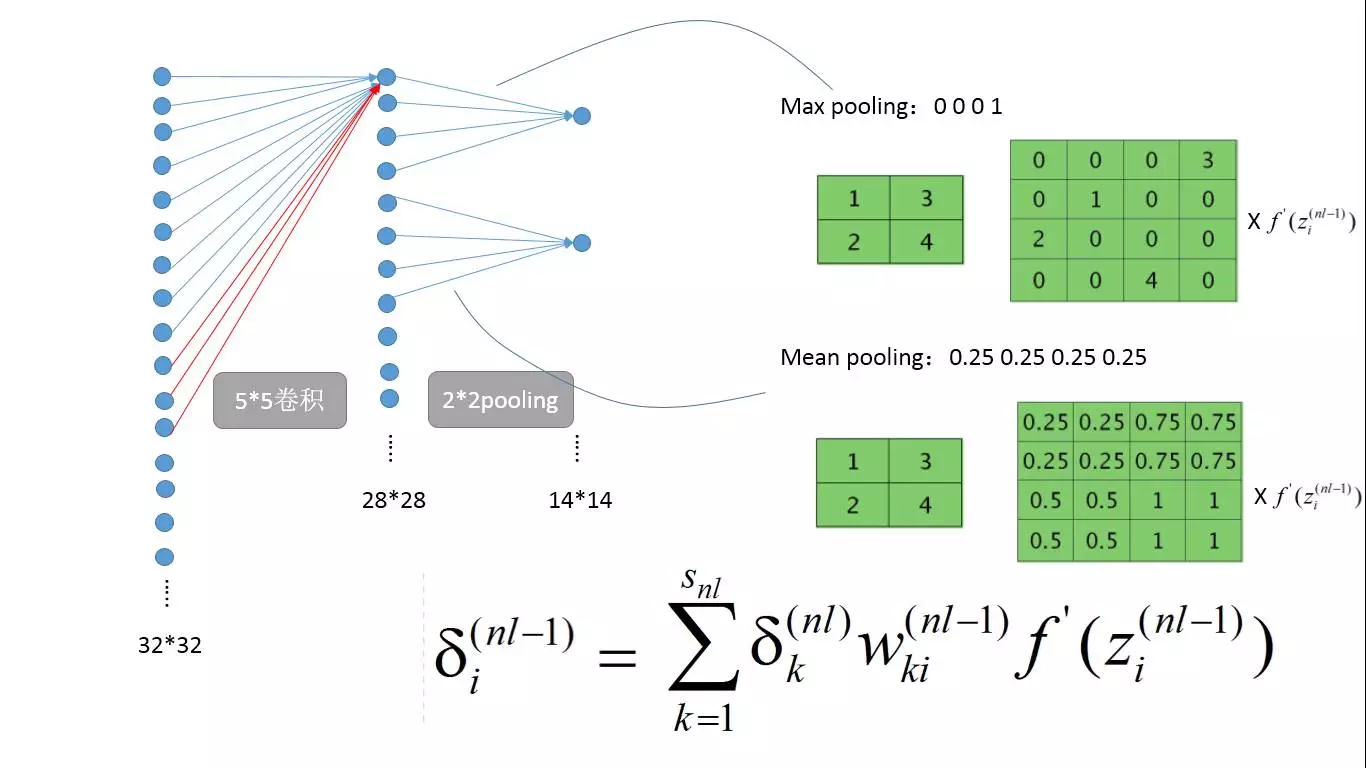（1）说明CNN是一种局部连接和权值共享的网络，这种网络可以看做是特殊的全连接网络，即不连接部分的权值为0。

（2）解释网上常见的pooling层向卷积层，以及卷积层向pooling层的残差反向传播的原理。

$z_i^{(l+1)}=w^{(l)}a^{(l)}+b^{(l)}$

$a^{(l+1)}=f(z^{(l+1)}$

$J(x^{(i)},y^{(i)};w,b)=\frac {1}{2}||h_{wb}(x^{(i)}-y^{(i)}||^2$

$J(w,b)=\frac {1}{m}\sum_{i=1}^{m}J(x^{(i)},y^{(i)};w,b)+\frac{\lambda }{2}\sum_{l=1}^{n_l-1}\sum_{i=1}^{s_l+1}\sum_{j}^{s_l}(w_{ij}^l)^2$

$w_{ij}^{(l)}=w{ij}^{(l)}-\frac{\partial J(w,b)}{\partial w_{ij}^{(l)}}$

$w_{ij}^{(l)}=w{ij}^{(l)}-\frac {1}{m}\sum_{i=1}^{m}\frac{\partial J(x^{(i)},y^{(i)};w,b)}{\partial w_{ij}^{(l)}}-\lambda w_{ji}^{(l)}$

$w_{ij}^{(l)}=w{ij}^{(l)}-\frac {1}{m}\sum_{i=1}^{m}\frac{\partial J(x^{(i)},y^{(i)};w,b)}{\partial z_{ij}^{(l+1)}}\frac{\partial z_{ij}^{(l+1)}}{\partial w_{ij}^{(l)}}-\lambda w_{ji}^{(l)}$

$Qz_{ij}^{(l+1)}=\sum_{k=1}^{sl}w_{ik}^{(l)}a_k^{(l)}+b_i^{(l)}$

$\therefore w_{ij}^{(l)}=w{ij}^{(l)}-\frac {1}{m}\sum_{i=1}^{m}\frac{\partial J(x^{(i)},y^{(i)};w,b)}{\partial z_{ij}^{(l+1)}}a_{j}^{(l)}-\lambda w_{ji}^{(l)}$

$\therefore b_i^{(l)}=b_i{(l)}-\frac {1}{m}\sum_{i=1}^{m}\frac{\partial J(x^{(i)},y^{(i)};w,b)}{\partial z_{ij}^{(l+1)}}$

$\delta _i^{(nl)}=\frac{\partial J(x,y;w,b)}{\partial z_i^{(nl)}}=\sum_{k=1}^{S_{nl}}(a_k^{(nl)}-y_k)\frac{\partial a_k^{(nl)}}{\partial z_i^{(nl)}}$

$=\sum_{k=1}^{S_{nl}}(a_k^{(nl)}-y_k)\frac{\partial (f(z_k^{(nl)}))}{\partial z_i^{(nl)}}=(a_i^{(nl)}-y_i)f'(z_i^{(nl)})$

$\delta_i^{(nl-1)}=\frac{\partial J(x,y;w,b)}{\partial z_i^{(nl-1)}}=\sum_{k=1}^{S_{nl}}(a_k^{(nl)}-y_k)\frac{\partial a_k^{(nl)}}{\partial z_i^{(nl-1)}}$

$=\sum_{k=1}^{S_{nl}}(a_k^{(nl)}-y_k)\frac{\partial (f(z_k^{(nl)}))}{\partial z_i^{(nl-1)}}=\sum_{k=1}^{S_{nl}}(a_k^{(nl)}-y_k)f'(z_k^{(nl)})\frac{\partial z_k{(nl)}}{\partial z_i^{(nl-1)}}$

$=\sum_{k=1}^{S_{nl}}(a_k^{(nl)}-y_k)f'(z_k^{(nl)})\frac{\partial (\sum_{r=1}^{S_{nl}}w_{kr}^{nl-1}f(z_r^{(nl-1)}+b_k^{(nl-1)})}{\partial z_i^{(nl-1)}}$

$=\sum_{k=1}^{S_{nl}}(a_k^{(nl)}-y_k)f'(z_k^{(nl)})w_{ki}^{(nl-1)}f'(z_i^{(nl-1)})$

$=\sum_{k=1}^{S_{nl}}\delta_k^{(nl-1)}w_{ki}^{(nl-1)}f'(z_i^{(nl-1)})$

$\therefore w_{ij}^{(l)}=w_{ij}^{(l)}-\frac{1}{m}\sum_{k=1}^{m}\delta_i^{(k,l+1)}a_j^{(k,l)}-\lambda w_{ij}^{(l)}$

$\therefore b_i^{(l)}=b_i^{(l)}-\frac{1}{m}\sum_{k=1}^{m}\delta_i^{(k,l+1)}$

$=\sum_{k=1}^{S_{nl}}\delta_k^{(nl)}w_{ki}^{(nl-1)}f'(z_i^{(nl-1)})$

*n的图像展开成列向量，长度就是n*n，那么后一层的节点个数就是（n-3+1)*(n-3+1)，也可以排成只有一列的列向量。这样的网络和MLP的网络是一样的，唯一的区别是非全连接，后一层的输入值之和前一层的九个节点有关系。我们只需要考虑CNN和MLP不同之处即可，相同的地方参考MLP的反向传播。显然不同之处在于CNN多了卷积计算和pooling。上图右边表示的是max pooling和mean pooling的反向传播示意图，以max pooling为例，其实是把残差直接给了前向传播pooling时值最大的节点。比如2*2的pooling，那么其实四个权重除了最大值的那个节点连接权重是1，其他三个都是0，那么再次参考残差传播的公式，权重为0，意味着反向残差为0，理解下面的公式，看看你就知道为啥是0了，w只有一个而且还是0，算出来当然是0。

$=\sum_{k=1}^{S_{nl}}\delta_k^{(nl)}w_{ki}^{(nl-1)}f'(z_i^{(nl-1)})$为啥卷积层的反向传播会是残差与卷积核的再卷积。要解释这个还得从残差的反向传播公式说起，上图分析的是红色节点影响了下一层的A11，A12....A33中的哪些节点，权重是多少。中间的星星图，就是具体影响。具体怎么得出这个图的，就是把做左图的3*3卷积核，往左边，右边，上面，下面，左上，左下，右上，右下，不动，共九个位置。看看红色部分影响到了后面中的哪些节点。根据残差反向传播公式，残差应该如下图计算：上图的上半部分就是完全按照计算公式得到的，下图就是传说中的用残差和卷积核做卷积，显然这两个式子是完全相等的。所以可以说，“莫名其妙”的残差卷积其实也是根据残差公式严格推导来的。多个卷积核的情况那还是一样的，多个卷积核与多个残差层逐一卷积后累加和。